# How to Easily Convert 60 Degrees Fahrenheit to Celsius: A Step-by-Step Guide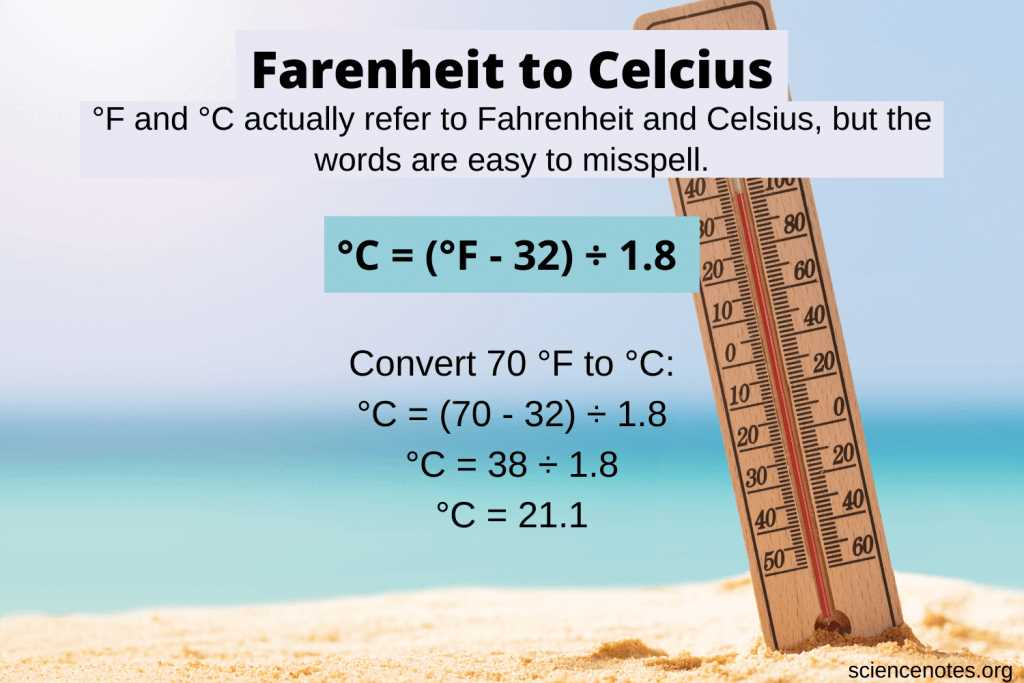When it comes to measuring temperature, there are two main systems used worldwide: Fahrenheit and Celsius. Fahrenheit is commonly used in the United States, while Celsius is the standard in most other countries. Converting between these two systems can be a bit confusing, but it’s actually quite simple once you understand the formula.

Today, we’ll focus on converting 60 degrees Fahrenheit to Celsius. To do this, we’ll use the formula: C = (F – 32) * 5/9. Let’s break it down step by step.

Step 1: Start with the Fahrenheit temperature you want to convert. In this case, we have 60 degrees Fahrenheit.

Step 2: Subtract 32 from the Fahrenheit temperature. In our case, 60 – 32 = 28.

Step 3: Multiply the result by 5/9. In our case, 28 * 5/9 = 15.555555555555555.

Step 4: Round the result to the desired number of decimal places. In this example, let’s round it to 2 decimal places. So, the final result is approximately 15.56 degrees Celsius.

By following these simple steps, you can easily convert 60 degrees Fahrenheit to Celsius. This conversion can be particularly useful when traveling or when dealing with temperature-sensitive tasks in different systems of measurement.

## Understanding the Fahrenheit and Celsius Scales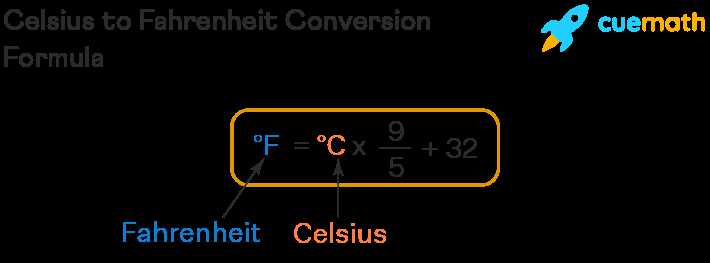The Fahrenheit and Celsius scales are two common temperature measurement systems used around the world. While both scales measure temperature, they have different reference points and increments. Understanding these scales is important for converting temperatures between them.

Fahrenheit Scale:

• The Fahrenheit scale is commonly used in the United States and a few other countries.
• The scale is based on a reference point of 32 degrees Fahrenheit (°F), which is the freezing point of water.
• The scale assigns the value of 212°F to the boiling point of water.
• The Fahrenheit scale has 180 equal divisions between the freezing and boiling points of water, resulting in smaller increments compared to the Celsius scale.
READ MORE  Discover the Perfect Shed for Living In: Your Ultimate Guide

Celsius Scale:

• The Celsius scale is used in most countries around the world.
• The scale is based on a reference point of 0 degrees Celsius (°C), which is the freezing point of water.
• The scale assigns the value of 100°C to the boiling point of water.
• The Celsius scale has 100 equal divisions between the freezing and boiling points of water, making it easier to understand and convert temperatures.

Converting Fahrenheit to Celsius:

To convert a temperature from Fahrenheit to Celsius, you can use the formula:

 Celsius = (Fahrenheit – 32) * 5/9

For example, to convert 60 degrees Fahrenheit to Celsius:

1. Subtract 32 from 60: 60 – 32 = 28
2. Multiply the result by 5/9: 28 * 5/9 = 15.56

Therefore, 60 degrees Fahrenheit is approximately equal to 15.56 degrees Celsius.

Understanding the Fahrenheit and Celsius scales is essential for accurately converting temperatures between the two systems. By knowing the reference points and increments of each scale, you can easily convert temperatures and understand the differences in temperature measurements.

### What is the Fahrenheit scale?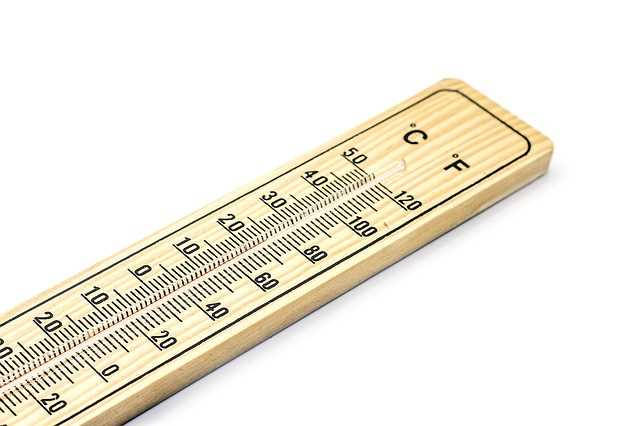The Fahrenheit scale is a temperature scale commonly used in the United States and a few other countries. It was developed by the Polish-German physicist Daniel Gabriel Fahrenheit in the early 18th century. The Fahrenheit scale is based on the freezing and boiling points of water, with 32 degrees Fahrenheit (°F) being the freezing point and 212 degrees Fahrenheit (°F) being the boiling point at standard atmospheric pressure.

The Fahrenheit scale is divided into equal intervals called degrees. Each degree on the Fahrenheit scale is equivalent to 1/180th of the interval between the freezing and boiling points of water. This means that there are 180 degrees between the freezing and boiling points of water on the Fahrenheit scale.

Converting temperatures from Celsius to Fahrenheit can be done using the following formula:

1. Multiply the temperature in Celsius by 9/5.
2. Add 32 to the result.

For example, to convert 60 degrees Celsius to Fahrenheit:

Celsius Fahrenheit
60 (60 × 9/5) + 32 = 140

So, 60 degrees Celsius is equivalent to 140 degrees Fahrenheit.

### What is the Celsius scale?

The Celsius scale is a temperature scale used in most countries around the world. It is based on the freezing and boiling points of water, with 0 degrees Celsius (°C) representing the freezing point and 100 degrees Celsius representing the boiling point. The Celsius scale is named after the Swedish astronomer Anders Celsius, who first proposed the scale in 1742.

READ MORE  Curtains as Closet Doors: A Stylish and Functional Solution

The Celsius scale is widely used for everyday temperature measurements, such as weather forecasts and household thermometers. It is a metric scale, which means it is based on units of 10. This makes conversions between different temperatures on the Celsius scale relatively simple.

To convert a temperature from Fahrenheit to Celsius, you can use the following formula:

Celsius = (Fahrenheit – 32) * 5/9

For example, if you want to convert 60 degrees Fahrenheit to Celsius, you would plug in the value of 60 into the formula:

Celsius = (60 – 32) * 5/9

Celsius = 28 * 5/9

Celsius = 15.56

So, 60 degrees Fahrenheit is approximately equal to 15.56 degrees Celsius.

The Celsius scale is widely used in scientific and academic settings, as well as in everyday life. It provides a standardized and easily understandable way to measure and compare temperatures.

## Converting 60 Degrees Fahrenheit to Celsius

To convert 60 degrees Fahrenheit to Celsius, you can use a simple formula. Follow the steps below:

2. Subtract 32 from the Fahrenheit temperature. In this case, 60 – 32 = 28.
3. Multiply the result by 5/9. In this case, 28 * 5/9 = 15.56.

Therefore, 60 degrees Fahrenheit is equal to approximately 15.56 degrees Celsius.

Remember that this is a general conversion formula and may not give you the exact value due to rounding. If you need a more precise conversion, you can use more decimal places in your calculations.

### Step 1: Subtract 32 from 60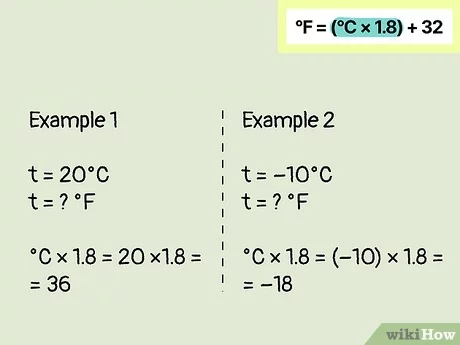To convert 60 degrees Fahrenheit to Celsius, you need to follow a simple conversion formula. The first step is to subtract 32 from the given temperature in Fahrenheit.

So, to convert 60 degrees Fahrenheit to Celsius, you subtract 32 from 60:

Step Calculation
1 60 – 32 = 28

After subtracting 32 from 60, you get 28. This means that 60 degrees Fahrenheit is equal to 28 degrees Celsius.

### Step 2: Multiply the result by 5

Now that we have converted 60 degrees Fahrenheit to Celsius, we need to multiply the result by 5.

READ MORE  Effective Ways to Catch a Rat: Tips and Techniques

The formula to convert Fahrenheit to Celsius is:

Celsius = (Fahrenheit – 32) * 5/9

In this case, we have already subtracted 32 from 60, which gives us 28. Now, we need to multiply 28 by 5:

Celsius = 28 * 5

Multiplying 28 by 5 gives us the final result:

Celsius = 140

So, 60 degrees Fahrenheit is equal to 140 degrees Celsius.

### Step 3: Divide the result by 9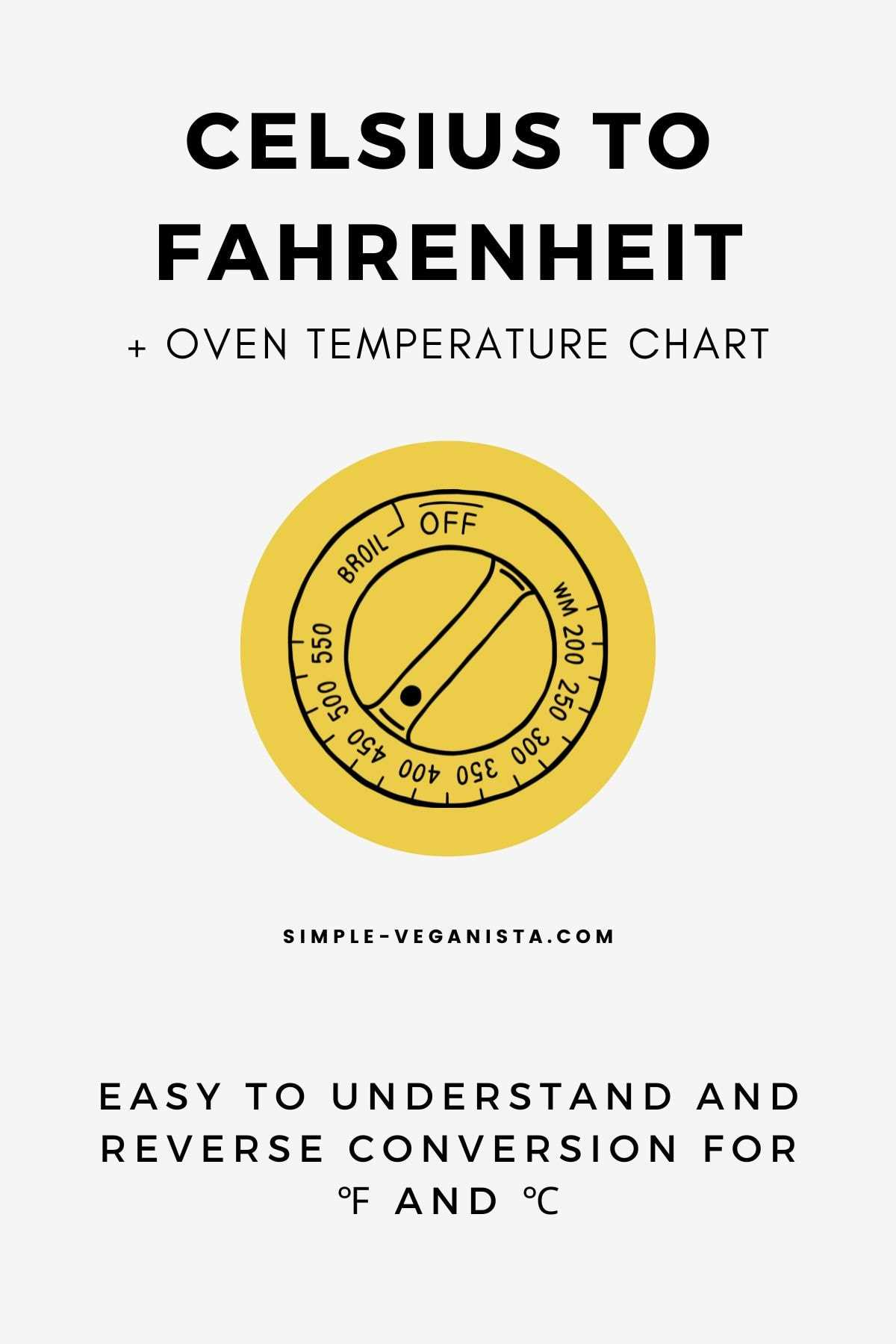After subtracting 32 degrees from the Fahrenheit temperature of 60, we obtain the result of 28 degrees. Now, we need to divide this result by 9 to convert it to Celsius.

Dividing 28 degrees by 9, we get the value of approximately 3.11 degrees Celsius.

Therefore, 60 degrees Fahrenheit is approximately equal to 3.11 degrees Celsius.